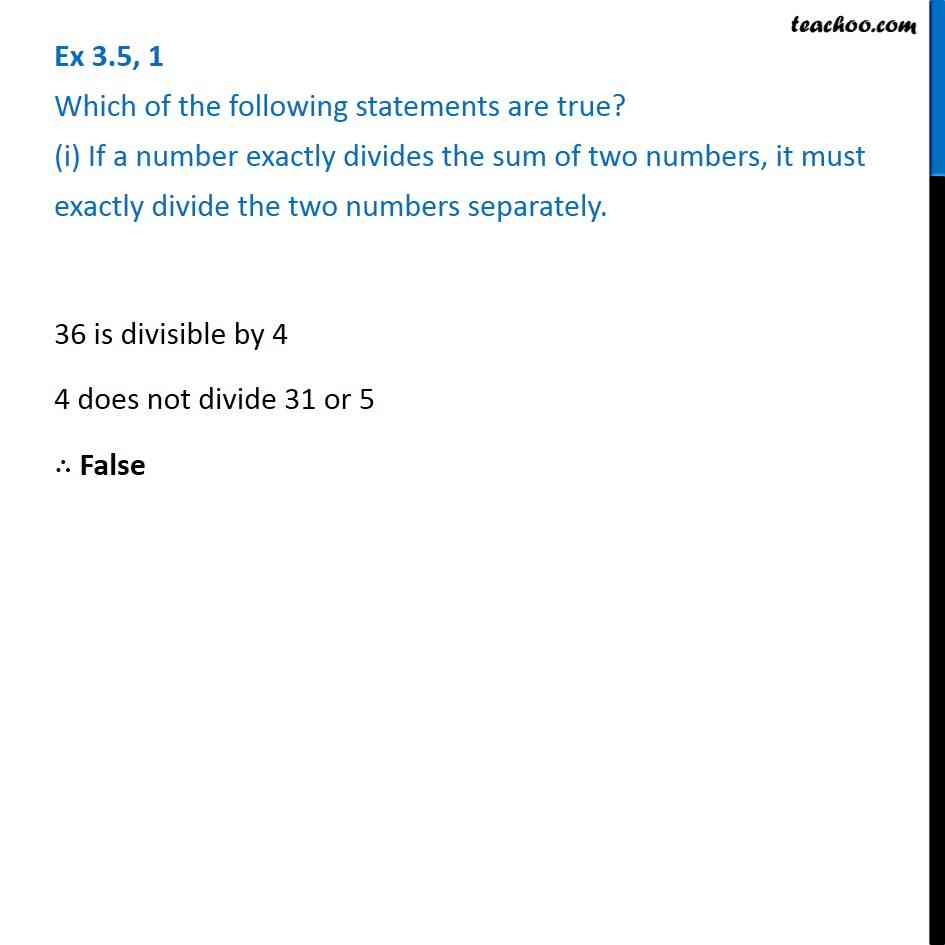Ex 3.5

Chapter 3 Class 6 Playing with Numbers
Serial order wiseLearn in your speed, with individual attention - Teachoo Maths 1-on-1 Class

### Transcript

Ex 3.5, 1 Which of the following statements are true? (i) If a number exactly divides the sum of two numbers, it must exactly divide the two numbers separately.36 is divisible by 4 4 does not divide 31 or 5 ∴ False